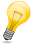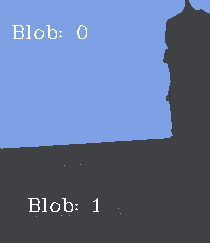## Chapter 3. Introduction to clustering, segmentation and connected components

In this tutorial we’ll create an application that demonstrates how an image can be broken into a number of regions. The process of separating an image into regions, or segments, is called segmentation. Segmentation is a widely studied area in computer vision. Researchers often try to optimise their segmentation algorithms to try and separate the objects in the image from the background.

To get started, create a new OpenIMAJ project using the Maven archetype, import it into your IDE, and delete the sample code from within the generated `main()` method of the `App` class. In the `main()` method, start by adding code to load an image (choose your own image):

`MBFImage input = ImageUtilities.readMBF(new URL("http://..."));`

To segment our image we are going to use a machine learning technique called clustering. Clustering algorithms automatically group similar things together. In our case, we’ll use a popular clustering algorithm called K-Means clustering to group together all the similar colours in our image. Each group of similar colours is known as a class. The K-means clustering algorithm requires you set the number of classes you wish to find a priori (i.e. beforehand).

K-means ClusteringK-Means initialises cluster centroids with randomly selected data points and then iteratively assigns the data points to their closest cluster and updates the centroids to the mean of the respective data points.

Colours in our input image are represented in RGB colour space; that is each pixel is represented as three numbers corresponding to a red, green and blue value. In order to measure the similarity of a pair of colours the distance between the colours in the colour space can be measured. Typically, the distance measured is the Euclidean distance. Unfortunately, distances in RGB colour space do not reflect what humans perceive as similar/dissimilar colours. In order to work-around this problem it is common to transform an image into an alternative colour space. The Lab colour space (pronounced as separate letters, L A B) is specifically designed so that the Euclidean distance between colours closely matches the perceived similarity of a colour pair by a human observer.

Euclidean DistanceThe Euclidean distance is the straight-line distance between two points. It is named after the "Father of Geometry", the Greek mathematician Euclid.

To start our implementation, we’ll first apply a colour-space transform to the image:

`input = ColourSpace.convert(input, ColourSpace.CIE_Lab);`

We can then construct the K-Means algorithm:

`FloatKMeans cluster = FloatKMeans.createExact(2);`

The parameter (2) is the number of clusters or classes we wish the algorithm to generate. We can optionally provide a second integer argument that controls the maximum number of iterations of the algorithm (the default is 30 iterations if we don't specify otherwise).

TipThere are a number of different static factory methods on the `FloatKMeans` class, as well as constructors that allow various flavours of the K-Means algorithm to be instantiated. In particular, the `FloatKMeans.createKDTreeEnsemble(int)` method creates an approximate K-means implementation using a technique based on an ensemble of KD-Trees. The approximate algorithm is much faster than the exact algorithm when there is very high-dimensional data; in this case, with only three dimensions, the approximate algorithm is not required. All the OpenIMAJ K-Means implementations are multithreaded and automatically takes advantage of all the processing power they can obtain by default. This behaviour can of course be controlled programatically however.

The `FloatKMeans` algorithm takes its input as an array of floating point vectors (`float[][]`). We can flatten the pixels of an image into the required form using the `getPixelVectorNative()` method:

`float[][] imageData = input.getPixelVectorNative(new float[input.getWidth() * input.getHeight()]);`

The K-Means algorithm can then be run to group all the pixels into the requested number of classes:

`FloatCentroidsResult result = cluster.cluster(imageData);`

Each class or cluster produced by the K-Means algorithm has an index, starting from 0. Each class is represented by its centroid (the average location of all the points belonging to the class). We can print the coordinates of each centroid:

```float[][] centroids = result.centroids;
for (float[] fs : centroids) {
System.out.println(Arrays.toString(fs));
}```

Now is a good time to test the code. Running it should print the (`L, a, b`) coordinates of each of the classes.

We can now use a `HardAssigner` to assign each pixel in our image to its respective class using the centroids learned during the `FloatKMeans`. This is a process known as classification. There are a number of different `HardAssigner`s, however, `FloatCentroidsResult` has a method called `defaultHardAssigner()` which will return an assigner fit for our purposes. `HardAssigner`s have a method called `assign()` which takes a vector (the `L, a, b` value of a single pixel) and returns the index of the class that it belongs to. We’ll start by creating an image that visualises the pixels and their respective classes by replacing each pixel in the input image with the centroid of its respective class:

```HardAssigner<float[],?,?> assigner = result.defaultHardAssigner();
for (int y=0; y<input.getHeight(); y++) {
for (int x=0; x<input.getWidth(); x++) {
float[] pixel = input.getPixelNative(x, y);
int centroid = assigner.assign(pixel);
input.setPixelNative(x, y, centroids[centroid]);
}
}```

We can then display the resultant image. Note that we need to convert the image back to RGB colour space for it to display properly:

```input = ColourSpace.convert(input, ColourSpace.RGB);
DisplayUtilities.display(input);```

Running the code will display an image that looks a little like the original image but with as many colours as there are classes.To actually produce a segmentation of the image we need to group together all pixels with the same class that are touching each other. Each set of pixels representing a segment is often referred to as a connected component. Connected components in OpenIMAJ are modelled by the `ConnectedComponent` class.

The `GreyscaleConnectedComponentLabeler` class can be used to find the connected components:

```GreyscaleConnectedComponentLabeler labeler = new GreyscaleConnectedComponentLabeler();
List<ConnectedComponent> components = labeler.findComponents(input.flatten());```

Note that the `GreyscaleConnectedComponentLabeler` only processes greyscale images (the `FImage` class) and not the colour image (`MBFImage` class) that we created. The `flatten()` method on `MBFImage` merges the colours into grey values by averaging their RGB values.

TipOpenIMAJ also contains a class called `ConnectedComponentLabeler` which can only be used on binary (pure black and white) `FImage`s.

The `ConnectedComponent` class has many useful methods for extracting information about the shape of the region. Lets draw an image with the components numbered on it. We’ll use the centre of mass of each region to position the number and only render numbers for regions that are over a certain size (50 pixels in this case):

```int i = 0;
for (ConnectedComponent comp : components) {
if (comp.calculateArea() < 50)
continue;
input.drawText("Point:" + (i++), comp.calculateCentroidPixel(), HersheyFont.TIMES_MEDIUM, 20);
}```

Finally, we can display the image with the labels:

`DisplayUtilities.display(input);`## 3.1. Exercises

### 3.1.1. Exercise 1: The PixelProcessor

Rather than looping over the image pixels using two for loops, it is possible to use a `PixelProcessor` to accomplish the same task:

```image.processInplace(new PixelProcessor<Float[]>() {
Float[] processPixel(Float[] pixel) {
...
}
});```

Can you re-implement the loop that replaces each pixel with its class centroid using a `PixelProcessor`?

What are the advantages and disadvantages of using a `PixelProcessor`?

### 3.1.2. Exercise 2: A real segmentation algorithm

The segmentation algorithm we just implemented can work reasonably well, but is rather naïve. OpenIMAJ contains an implementation of a popular segmentation algorithm called the `FelzenszwalbHuttenlocherSegmenter`.

Try using the `FelzenszwalbHuttenlocherSegmenter` for yourself and see how it compares to the basic segmentation algorithm we implemented. You can use the `SegmentationUtilities.renderSegments()` static method to draw the connected components produced by the segmenter.